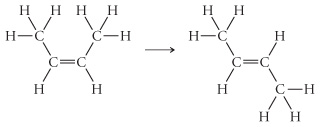# Problem: cis-2-Butene isomerizes to trans-2-butene via the following reaction .If the energy for isomerization came from light, what minimum frequency of light would be required?

###### FREE Expert Solution

We can look up the bond energy for a C=C bond in textbooks or online: 611 kJ/mol. This bond energy includes both σ and π bonds. The bond energy for a C–C σ bond is 347 kJ/mol.

Isomerization requires breaking only the π bond. This means the bond energy of a C–C π bond is:

611 kJ/mol – 347 kJ/mol = 264 kJ/mol

Recall that Avogadro's Number gives us 6.022 × 1023 molecules in 1 mole. This means the energy needed to break a C–C π bond in one molecule of cis-2-butene is:93% (388 ratings)###### Problem Details

cis-2-Butene isomerizes to trans-2-butene via the following reaction.

If the energy for isomerization came from light, what minimum frequency of light would be required?